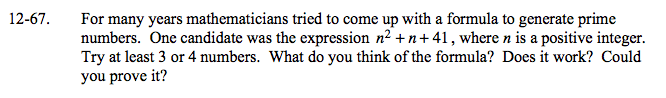### Home > A2C > Chapter 12 > Lesson 12.1.4 > Problem12-67

12-67.

For many years mathematicians tried to come up with a formula to generate prime numbers. One candidate was the expression n2 + n + 41, where n is a positive integer. Try at least 3 or 4 numbers. What do you think of the formula? Does it work? Could you prove it? Homework Help ✎Try 1, 2, 3 and 4. Does it work?

Try 41. Does it work?